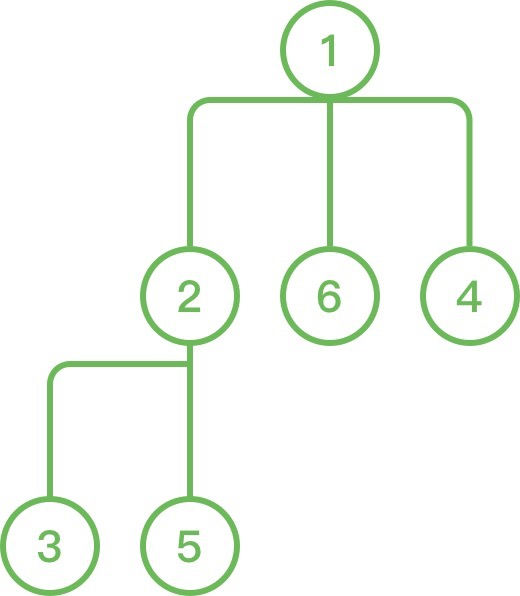# 5. 发 LeetCoin¶

## 1、题目描述¶

1. 团队只有一个负责人，编号为1。除了该负责人外，每个人有且仅有一个领导（负责人没有领导）；
2. 不存在循环管理的情况，如A管理B，B管理C，C管理A。

1. 给团队的一个成员（也可以是负责人）发一定数量的LeetCoin
2. 给团队的一个成员（也可以是负责人），以及他/她管理的所有人（即他/她的下属、他/她下属的下属，……），发一定数量的LeetCoin
3. 查询某一个成员（也可以是负责人），以及他/她管理的所有人被发到的LeetCoin之和。

1. N表示团队成员的个数（编号为1～N，负责人为1）；

2. leadership是大小为(N - 1) * 2的二维数组，其中每个元素[a, b]代表ba的下属；

operations是一个长度为Q的二维数组，代表以时间排序的操作，格式如下：

1. operations[i] = 1: 代表第一种操作，operations[i]代表成员的编号，operations[i]代表LeetCoin的数量；
2. operations[i] = 2: 代表第二种操作，operations[i]代表成员的编号，operations[i]代表LeetCoin的数量；
3. operations[i] = 3: 代表第三种操作，operations[i]代表成员的编号；

输入：N = 6, leadership = [[1, 2], [1, 6], [2, 3], [2, 5], [1, 4]], operations = [[1, 1, 500], [2, 2, 50], [3, 1], [2, 6, 15], [3, 1]]1. 1 <= N <= 50000
2. 1 <= Q <= 50000
3. operations[i] != 3 时，1 <= operations[i] <= 5000

## 2、解题思路¶

• 题目关键点在于需要不断的更新当前节点管理的硬币数量
• 采用字典同时保存子节点，父节点
• 并且使用数组保存当前节点管理的节点总数，这样在更新子节点硬币数量的时候能够直接计算出来
from typing import List

from collections import defaultdict

class Solution:
def bonus(self, n: int, leadership: List[List[int]], operations: List[List[int]]) -> List[int]:
max_num = 1000000007

mapping = defaultdict(list)
father = defaultdict(int)

score = {k: 0 for k in range(1, n + 1)}
# 子节点数量
sub_count =  * n

def dfs_sub(k):
count = 1
for nex in mapping[k]:
count += dfs_sub(nex)

sub_count[k - 1] = count
return count

# 更新子节点数量
dfs_sub(1)

def dfs(k, coin):
for nex in mapping[k]:
dfs(nex, coin)
score[k] = (score[k] + sub_count[k - 1] * coin) % max_num

ans = []

for op in operations:

if len(op) == 3:
if op == 1:
t1 = op
score[t1] += op
while t1 in father:
t1 = father[t1]
score[t1] += op

elif op == 2:
dfs(op, op)
cur_score = sub_count[op - 1] * op
t1 = op
while t1 in father:
t1 = father[t1]
score[t1] = (score[t1] + cur_score) % max_num

else:

ans.append(score[op])
return ans